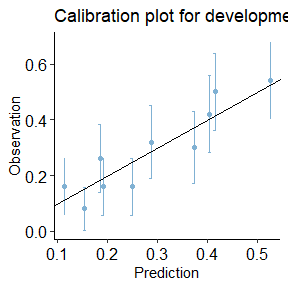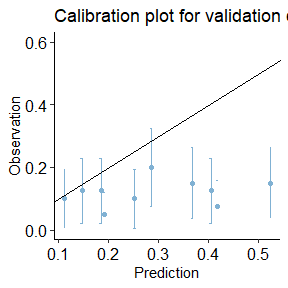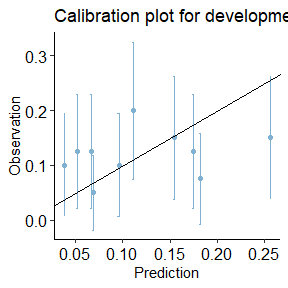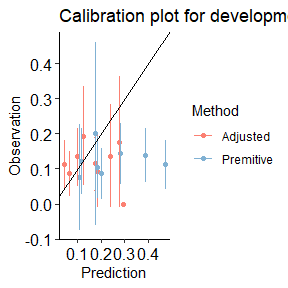library(predtools)
library(magrittr)
library(dplyr)
library(ggplot2)

In clinical prediction modeling, model updating refers to the practice of modifying a prediction model before it is used in a new setting to improve its performance. One of the simplest updating methods for risk predication models is a fixed odds-ratio transformation of predicted risks to improve the model’s calibration-in-the-large.

interceptAdj function uses an approximate equation for recovering the conditional odds-ratio from the observed mean and predicted variance of risks in validation and development sets, respectively.

A step-by-step guide.

Imagine the variable y indicates risk of disease recurrence in a unit of time. We have a prediction model that quantifies this risk given a patient’s age, disease severity level, sex, and whether the patient has a comorbidity.

The package comes with two exemplary datasets. dev_data and val_data. We use the dev_data as the development sample and the val_data as the external validation sample.

Model updating matters when there is a considerable difference between mean of the observed risks in development and validation sets. The average of y in the above two datasets are almost identical. Therefore, to have a meaningful scenario, we create a secondary (arbitrary) outcome (y_alt) in val_data with a lower average (by ~ 50%).

data(dev_data)
data(val_data)
y y_alt
Min. :0.0000 Min. :0.00
1st Qu.:0.0000 1st Qu.:0.00
Median :0.0000 Median :0.00
Mean :0.2975 Mean :0.12
3rd Qu.:1.0000 3rd Qu.:0.00
Max. :1.0000 Max. :1.00

dev_data has 500 rows. val_data has 400 rows.

Here are the first few rows of dev_data:

age severity sex comorbidity y
55 0 0 1 1
52 1 0 0 0
63 0 0 1 0
61 1 1 1 1
58 0 1 0 0
54 1 0 0 1
45 0 0 0 0

We use the development data to fit a logistic regression model as our risk prediction model:

summary(reg)
#>
#> Call:
#> glm(formula = y ~ sex + age + severity + comorbidity, family = binomial(link = "logit"),
#>     data = dev_data)
#>
#> Deviance Residuals:
#>     Min       1Q   Median       3Q      Max
#> -1.2904  -0.8272  -0.6373   1.1570   2.1050
#>
#> Coefficients:
#>              Estimate Std. Error z value Pr(>|z|)
#> (Intercept) -1.728929   0.565066  -3.060  0.00222 **
#> sex          0.557178   0.223631   2.492  0.01272 *
#> age          0.005175   0.010654   0.486  0.62717
#> severity    -0.557335   0.227587  -2.449  0.01433 *
#> comorbidity  1.091936   0.209944   5.201 1.98e-07 ***
#> ---
#> Signif. codes:  0 '***' 0.001 '**' 0.01 '*' 0.05 '.' 0.1 ' ' 1
#>
#> (Dispersion parameter for binomial family taken to be 1)
#>
#>     Null deviance: 602.15  on 499  degrees of freedom
#> Residual deviance: 560.41  on 495  degrees of freedom
#> AIC: 570.41
#>
#> Number of Fisher Scoring iterations: 4

Given this, our risk prediction model can be written as:

$$\bf{ logit(p)=-1.7289+0.5572*sex+0.0052*age-0.5573*severity+1.0919*comorbidity}$$.

First, let’s see the calibration plot in development and validation datasets. We use calibration_plot from our package to create calibration plots.

dev_data$pred <- predict.glm(reg, type = 'response') val_data$pred <- predict.glm(reg, newdata = val_data, type = 'response')

calibration_plot(data = dev_data, obs = "y", pred = "pred", title = "Calibration plot for development data")
#> $calibration_plotcalibration_plot(data = val_data, obs = "y_alt", pred = "pred", y_lim = c(0, 0.6), title = "Calibration plot for validation data") #>$calibration_plotTo adjust the predicted risks for the validation set, we estimate the correction factor by using function odds_adjust:

odds_correction_factor <- odds_adjust(p0 = mean(dev_data$y), p1 = mean(val_data$y_alt), v = var(dev_data$pred)) odds_correction_factor #>  0.3116119 We can now recalibrate the predictions and reproduce the calibration plot for the validation set. dev_data$pred <- predict.glm(reg, type = 'response')
val_data$pred <- predict.glm(reg, newdata = val_data, type = 'response') val_data$odds_adj <- (val_data$pred / (1 - val_data$pred)) * odds_correction_factor
val_data$pred_adj <- val_data$odds_adj / (1 + val_data$odds_adj) val_data$id <- c(1 : nrow(val_data))
val_data_long <- reshape(data = val_data, direction = "long", varying = c("pred", "pred_adj"), v.name = "preds",
idvar = "id", timevar = "Method", times = c("Premitive", "Adjusted"))

calibration_plot(data = val_data, obs = "y_alt", pred = "pred_adj",
title = "Calibration plot for development data - after recalibration")
#> $calibration_plotcalibration_plot(data = val_data_long, obs = "y_alt", pred = "preds", group = "Method", title = "Calibration plot for development data - before and after recalibration") #>$calibration_plot